electricity. The following is a summary of the important points in the chapter. A BASIC ELECTRIC CIRCUIT consists of a source of electrical energy connected to a load. The load uses the energy and changes it to a useful form."> Summary - Page 1-43Custom SearchElectrical firesMatter, Energy, and ElectricityRules for series DC circuitsSUMMARY

With the completion of this chapter you have gained a basic understanding of dc circuits. The information you have learned will provide you with a firm foundation for continuing your study of electricity. The following is a summary of the important points in the chapter.

A BASIC ELECTRIC CIRCUIT consists of a
source of electrical energy connected to a load. The load uses the energy
and changes it to a useful form.A SCHEMATIC DIAGRAM is a "picture" of a circuit, which uses symbols to represent components. The space required to depict an electrical or electronic circuit is greatly reduced by the use of a schematic.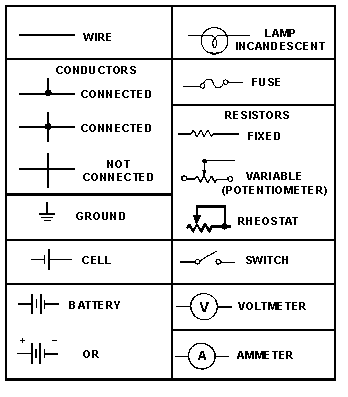VOLTAGE (E) is the electrical force or pressure operating in a circuit.

AN AMPERE (A) represents the current flow produced by one volt working across one ohm of resistance.

RESISTANCE (R) is the opposition to current. It is measured in ohms (Ω). One ohm of resistance will limit the current produced by one volt to a level of one ampere.

THE OHM'S FORMULA can be transposed to find one of the values in a circuit if the other two values are known. You can transpose the Ohm's law formula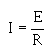mathematically, or you can use the Ohm's law figure to determine the mathematical relationship between R, E, and I.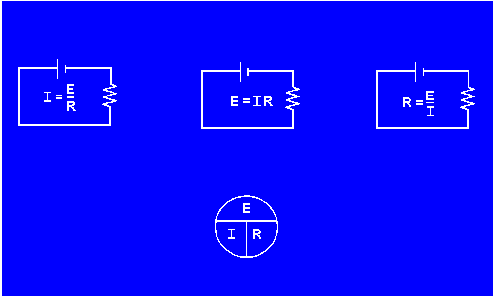GRAPHICAL ANALYSIS of the relationship between R, E, and I can be studied by plotting these quantities on a graph. Such a graph is useful for observing the characteristics of an electrical device.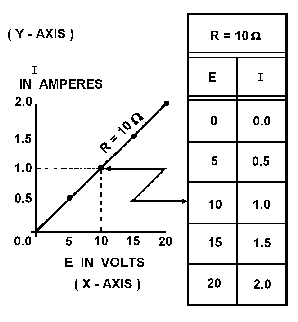POWER is the rate of doing work per unit of time. The time required to perform a given amount of work will determine the power expended. As a formula, P = E x I, where P = power in watts, E = voltage in volts, and I = current in amperes.

THE FOUR BASIC ELECTRICAL QUANTITIES are P, I, E, R. Any single unknown quantity can be expressed in terms of any two of the other known quantities. The formula wheel is a simple representation of these relationships.POWER RATING in watts indicates the rate at which a device converts electrical energy into another form of energy. The power rating of a resistor indicates the maximum power the resistor can withstand without being destroyed.

POWER USED by an electrical device is measured in watt-hours. One watt-hour is equal to one watt used continuously for one hour.

THE EFFICIENCY of an electrical device is equal to the electrical power converted into useful energy divided by the electrical power supplied to the device.HORSEPOWER is a unit of measurement often used to rate electrical motors. It is a unit of work. One horsepower is equal to 746 watts.

A SERIES CIRCUIT is defined as a circuit that has only one path for current flow.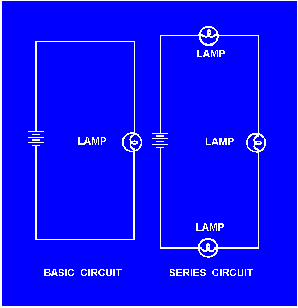Integrated Publishing, Inc. - A (SDVOSB) Service Disabled Veteran Owned Small Business